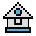Home

# COS Function

#### Purpose:

To return the cosine of the range of x.

#### Syntax:

`COS(x)`

x must be the radians. COS is the trigonometric cosine function. To convert from degrees to radians, multiply by π/180.

COS(x) is calculated in single precision unless the /d switch is used when GW-BASIC is executed.

Example 1:

```10 X=2*COS(.4)
20 PRINT X
RUN
Ā1.842122```

Example 2:

```10 PI=3.141593
20 PRINT COS(PI)
30 DEGREES=180